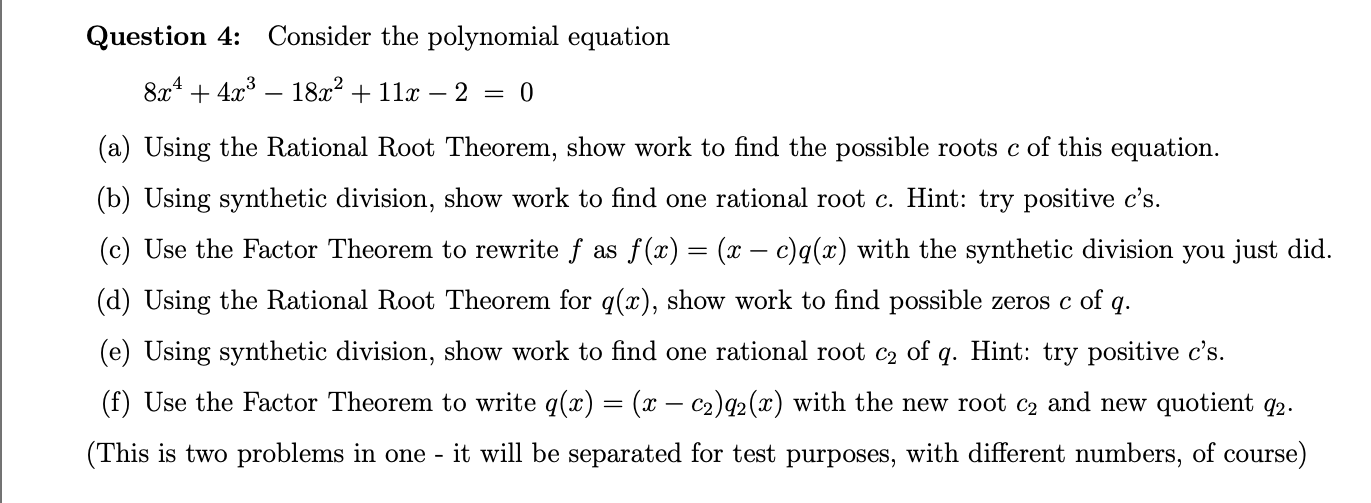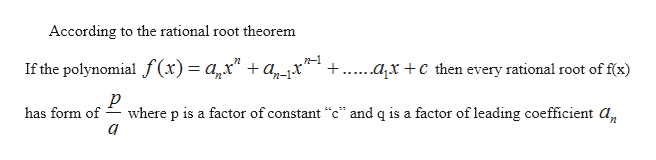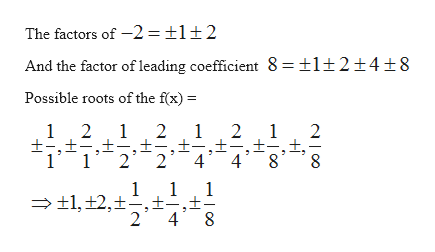# Question 4:Consider the polynomial equation8x44318a211- 2 =0(a) Using the Rational Root Theorem, show work to find the possible roots c of this equation(b) Using synthetic division, show work to find one rational root c. Hint: try positive c's.(c) Use the Factor Theorem to rewrite f as f (x) = (x - c)q(x) with the synthetic division you just did.(d) Using the Rational Root Theorem for q(x), show work to find possible zeros c of q(e) Using synthetic division, show work to find one rational root c2 of q. Hint: try positive c's.(f) Use the Factor Theorem to write q(x) = (x - c2)q2(x) with the new root c2 and new quotient q2.(This is two problems in oneit will be separated for test purposes, with different numbers, of course)

Question
1 viewshelp_outlineImage TranscriptioncloseQuestion 4: Consider the polynomial equation 8x443 18a211- 2 = 0 (a) Using the Rational Root Theorem, show work to find the possible roots c of this equation (b) Using synthetic division, show work to find one rational root c. Hint: try positive c's. (c) Use the Factor Theorem to rewrite f as f (x) = (x - c)q(x) with the synthetic division you just did. (d) Using the Rational Root Theorem for q(x), show work to find possible zeros c of q (e) Using synthetic division, show work to find one rational root c2 of q. Hint: try positive c's. (f) Use the Factor Theorem to write q(x) = (x - c2)q2(x) with the new root c2 and new quotient q2. (This is two problems in one it will be separated for test purposes, with different numbers, of course) fullscreen
check_circle

Step 1

Given that the polynomial

Step 2
1. Find out the factors using rational root theoremhelp_outlineImage TranscriptioncloseAccording to the rational root theorem n-1 ..axc then every rational root of f(x) If the polynomial f(x)= a,x" +a^x' р has form of where p is a factor of constant "c" and q is a factor of leading coefficient a, а fullscreen
Step 3

Here the constant is -2 and le...help_outlineImage TranscriptioncloseThe factors of -2 1±2 And the factor of leading coefficient 8 =±1±2±4±8 Possible roots of the f(x) 1 2 1 2 1 2 1 2 1 1 1 tl,,24 8 fullscreen

### Want to see the full answer?

See Solution

#### Want to see this answer and more?

Solutions are written by subject experts who are available 24/7. Questions are typically answered within 1 hour.*

See Solution
*Response times may vary by subject and question.
Tagged in

### Polynomials